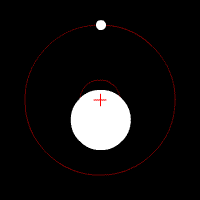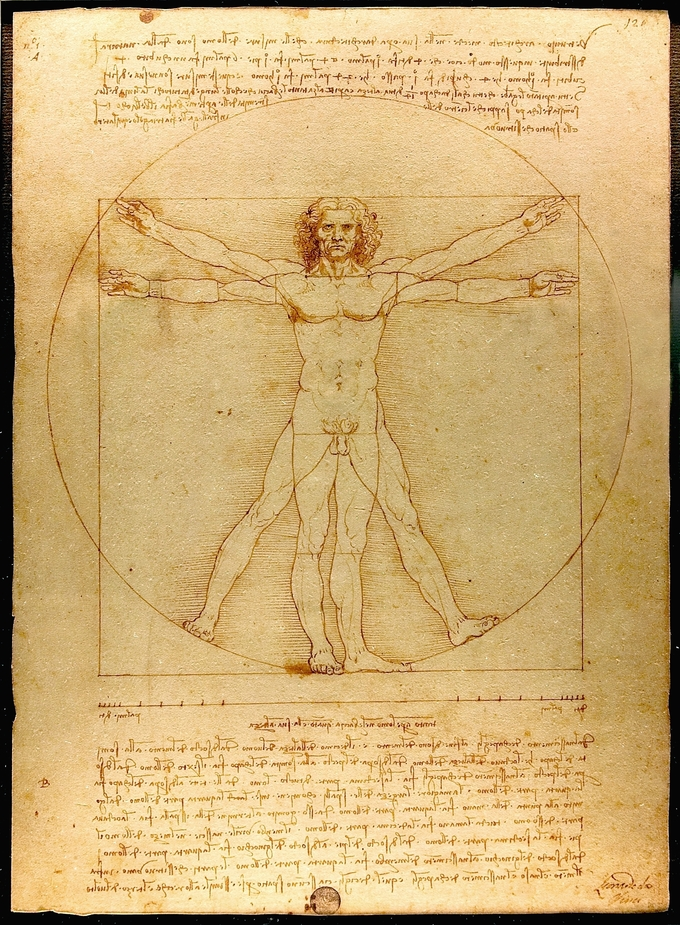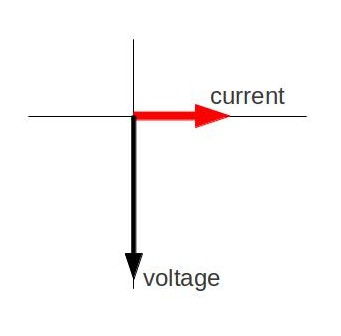## Locating the Center of Mass

The position of COM is mass weighted average of the positions of particles. Mathematically, it is given as $\bf{\text{r}}_{\text{COM}} = \frac{\sum_\text{i} \text{m}_\text{i} \bf{\text{r}}_\text{i}}{\text{M}}$.

### Learning Objectives

Identify the center of mass for an object with continuous mass distribution

### Key Takeaways

#### Key Points

• The center of mass (COM) is a statement of spatial arrangement of mass (i.e. distribution of mass within the system).
• The experimental determination of the center of mass of a body uses gravity forces on the body and relies on the fact that in the parallel gravity field near the surface of the earth the center of mass is the same as the center of gravity.
• For a 2D object, an experimental method for locating the center of mass is to suspend the object from two locations and to drop plumb lines from the suspension points. The intersection of the two lines is the center of mass.

#### Key Terms

• plumb line: A cord with a weight attached, used to produce a vertical line.

In the previous atom on “Center of Mass and Translational Motion,” we learned why the concept of center of mass (COM) helps solving mechanics problems involving a rigid body. Here, we will study the rigorous definition of COM and how to determine the location of it.

### Definition

The center of mass is a statement of spatial arrangement of mass (i.e. distribution of mass within the system). The position of COM is given a mathematical formulation which involves distribution of mass in space:

$\textbf{\text{r}}_{\text{COM}} = \frac{\sum_\text{i} \text{m}_\text{i} \textbf{\text{r}}_\text{i}}{\text{M}}$,

where rCOM and ri are vectors representing the position of COM and i-th particle respectively, and M and mi are the total mass and mass of the i-th particle, respectively. This mean means that position of COM is mass weighted average of the positions of particles.

### Object with Continuous Mass Distribution

If the mass distribution is continuous with the density ρ(r) within a volume V, the position of COM is given as

$\textbf{r}_{\text{COM}} = \frac {1}{\text{M}} \int_\text{V}\rho(\mathbf{\text{r}}) \mathbf{\text{r}} \text{dV}$,

where M is the total mass in the volume. If a continuous mass distribution has uniform density, which means ρ is constant, then the center of mass is the same as the center of the volume.

### Locating the Center of Mass

The experimental determination of the center of mass of a body uses gravity forces on the body and relies on the fact that in the parallel gravity field near the surface of Earth the center of mass is the same as the center of gravity.

The center of mass of a body with an axis of symmetry and constant density must lie on this axis. Thus, the center of mass of a circular cylinder of constant density has its center of mass on the axis of the cylinder. In the same way, the center of mass of a spherically symmetric body of constant density is at the center of the sphere. In general, for any symmetry of a body, its center of mass will be a fixed point of that symmetry.

In two dimensions: An experimental method for locating the center of mass is to suspend the object from two locations and to drop plumb lines from the suspension points. The intersection of the two lines is the center of mass.Plumb Line Method for Center of Mass: Suspend the object from two locations and to drop plumb lines from the suspension points. The intersection of the two lines is the center of mass.

In three dimensions: By supporting an object at three points and measuring the forces that resist the weight of the object, COM of the three-dimensional coordinates of the center of mass can be determined.

## Motion of the Center of Mass

We can describe the translational motion of a rigid body as if it is a point particle with the total mass located at the COM—center of mass.

### Learning Objectives

Derive the center of mass for the translational motion of a rigid body

### Key Takeaways

#### Key Points

• The total mass times the acceleration of the center of mass equals the sum of external forces.
• For the translational motion of a rigid body with mass M, Newton’s 2nd law applies as if we are describing the motion of a point particle (with mass M) under the influence of the external force.
• When there is no external force, the center of mass momentum is conserved.

#### Key Terms

• rigid body: An idealized solid whose size and shape are fixed and remain unaltered when forces are applied; used in Newtonian mechanics to model real objects.
• center of mass: The center of mass (COM) is the unique point at the center of a distribution of mass in space that has the property that the weighted position vectors relative to this point sum to zero.

We can describe the translational motion of a rigid body as if it is a point particle with the total mass located at the center of mass (COM). In this Atom. we will prove that the total mass (M) times the acceleration of the COM (aCOM), indeed, equals the sum of external forces. That is,

$\text{M} \cdot \textbf{a}_{\text{COM}} = \sum \textbf{F}_{\text{ext}}$.

You can see that the Newton’s 2nd law applies as if we are describing the motion of a point particle (with mass M) under the influence of the external force.

### Derivation

From the definition of the center of mass,

$\textbf{r}_{\text{COM}} = \frac{\sum_\text{i} \text{m}_\text{i} \textbf{r}_\text{i}}{\text{M}}$,

we get $\text{M} \cdot \textbf{a}_{\text{COM}} = \sum \text{m}_\text{i} \textbf{a}_\text{i}$ by taking time derivative twice on each side.

Note that $\sum \text{m}_\text{i} \textbf{a}_\text{i} = \sum \textbf{F}_\text{i}$.

In a system of particles, each particle may feel both external and internal forces. Here, external forces are forces from external sources, while internal forces are forces between particles in the system. Since the sum of all internal forces will be 0 due to the Newton’s 3rd law,

$\sum \textbf{F}_\text{i} = \sum \textbf{F}_{\text{i}, \text{ext}}$. Therefore, we get $\text{M} \cdot \textbf{a}_{\text{COM}} = \sum \textbf{F}_{\text{ext}}$.

For example, when we confine our system to the Earth and the Moon, the gravitational force due to the Sun would be external, while the gravitational force on the Earth due to the Moon (and vice versa) would be internal. Since the gravitational forces between the Earth and the Moon are equal in magnitude and opposite in direction, they will cancel out each other in the sum (see ).COM of the Earth and Moon: Earth and Moon orbiting a COM inside the Earth. The red cross represents the COM of the two-body system. The COM will orbit around the Sun as if it is a point particle.

### Corollary

When there is no external force, the COM momentum is conserved.

Proof: Since there is no external force, $\text{M} \cdot \bf{\text{a}}_{\text{COM}} = 0$. Therefore,

$\text{M} \cdot \bf{\text{v}}_{\text{COM}} = \text{constant}$.

## Center of Mass of the Human Body

The center of mass (COM) is an important physical concept—it is the point about which objects rotate.

### Learning Objectives

Estimate the COM of a given object

### Key Takeaways

#### Key Points

• Although a human body has complicated features, the location of the center of mass (COM) could be a good indicator of the body proportions.
• We can measure the location of COM with two scales and a wooden beam. The linear and rotational equations of motion gives us the location.
• The center of mass of the human body depends on the gender and the position of the limbs. In a standing posture, it is typically about 10 cm lower than the navel, near the top of the hip bones.

#### Key Terms

• center of mass: The center of mass (COM) is the unique point at the center of a distribution of mass in space that has the property that the weighted position vectors relative to this point sum to zero.
• torque: A rotational or twisting effect of a force; (SI unit newton-meter or Nm; imperial unit foot-pound or ft-lb)

The center of mass (COM) is an important physical concept. It is the point on an object at which the weighted relative position of the distributed mass sums to zero—the point about which objects rotate.

Human proportions have been important in art, measurement, and medicine (a well known drawing of the human body is seen in ). Although the human body has complicated features, the location of the center of mass (COM) could be a good indicator of body proportions. The center of mass of the human body depends on the gender and the position of the limbs. In a standing posture, it is typically about 10 cm lower than the navel, near the top of the hip bones. In this Atom, we will learn how to measure the COM of a human body.Leonardo da Vinci’s “The Vitruvian Man”: Vitruvian Man: A drawing created by Leonardo da Vinci. The drawing is based on the correlations of ideal human proportions with geometry described by the ancient Roman architect Vitruvius in Book III of his treatise De Architectura.

First, let’s take two scales and a wooden beam (H meter long), long enough to contain the entire body of the subject. Put the scales H meters apart, and place the beam across the scales, as illustrated in. Now, let the subject lie on the beam. Make sure that his/her heels are aligned with one end of the beam. Measure the readings (F1, F2) on the scale.The COM of a Human Body: This figure demonstrates measuring the COM of a human body.

The system (person+beam) has three external forces: gravity on the subject (FCM), and normal forces from the scales F1 and F2. The equation of motion for force (F=ma) will give us:

$\text{F}_1 + \text{F}_2 = \text{Mg}$,

where M is mass of the subject. (We assume that the wooden beam has no mass. ) This equation doesn’t provide all the information to locate the COM. However, the equation of motion for torque $( \tau = \text{I}\alpha)$ helps.

Since the net torque of the system is zero (hence no rotational acceleration),

$\text{h} \text{F}_2 - (\text{H}-\text{h}) \text{F}_1 = 0$. (h: COM height)

The COM is chosen as the origin for the torque. Therefore, gravity contributes nothing as a torque. Solving for h and using the equation of motion for force, we get

$\text{h} = \frac{\text{HF}_1}{\text{Mg}}$.

## Center of Mass and Translational Motion

The COM (center of mass) of a system of particles is a geometric point that assumes all the mass and external force(s) during motion.

### Learning Objectives

Support the presence of COM in three dimensional bodies in motion

### Key Takeaways

#### Key Points

• In a motion of a rigid body, different parts of the body have different motions.This means that these bodies may not behave like a point particle.
• There is a characteristic geometric point of the three dimensional body in motion. This point behaves as a particle, and is known as center of mass, abbreviated COM. COM appears to carry the whole mass of the body. All external forces appear to apply at COM.
• To describe the motion of a rigid body (with possibly a complicated geometry), we separate the translational part of the motion from the rotational part.

#### Key Terms

• rigid body: An idealized solid whose size and shape are fixed and remain unaltered when forces are applied; used in Newtonian mechanics to model real objects.
• point particle: An idealization of particles heavily used in physics. Its defining feature is that it lacks spatial extension, meaning that geometrically the particle is equivalent to a point.

### Introduction: COM, Linear Momentum, and Collisions

Our study of motion has been limited up to this point. We have referred to particle, object and body in the same way. We considered that actual three dimensional rigid bodies move such that all constituent particles had the same motion (i.e., same trajectory, velocity and acceleration). By doing this, we have essentially considered a rigid body as a point particle.

### Center of Mass (COM)

An actual body, however, can move differently than this simplified paradigm. Consider a ball rolling down an incline plane or a stick thrown into air. Different parts of a body have different motions. While translating in the air, the stick rotates about a moving axis, as shown in. This means that such bodies may not behave like a point particle, as earlier suggested.Forces on the COM: Left: The force appears to operate on the COM is “mgsinθ. Right: The force appears to operate on the COM is “mg”.

Describing motions of parts or particles that have different motions would be quite complicated to do in an integrated manner. However, such three dimensional bodies in motion have one surprising, simplifying characteristic—a geometric point that behaves like a particle. This point is known as center of mass, abbreviated COM (the mathematical definition of COM will be introduced in the next Atom on “Locating the Center of Mass”). It has the following two characterizing aspects:

• The center of mass appears to carry the whole mass of the body.
• At the center of mass, all external forces appear to apply.

Significantly, the center of a ball (the COM of a rolling ball) follows a straight linear path; whereas the COM of a stick follows a parabolic path (as shown in the figure above). Secondly, the forces appear to operate on the COMs in two cases (“mgsinθ and “mg”) as if they were indeed particle-like objects. This concept of COM, therefore, eliminate the complexities otherwise present in attempting to describe motions of rigid bodies.

### Describing Motion in a Rigid Body

We can describe general motion of an object (with mass m) as follows:

• We describe the translational motion of a rigid body as if it is a point particle with mass m located at COM.
• Rotation of the particle, with respect to the COM, is described independently.

We “separate” the translational part of the motion from the rotational part. By introducing the concept of COM, the translational motion becomes that of a point particle with mass m. This simplifies significantly the mathematical complexity of the problem.